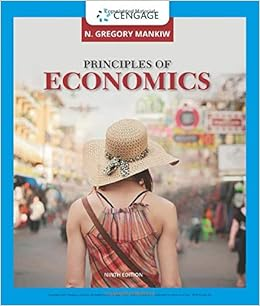# If a bank that is subject to a 10 percent required

• Test Prep
• DeaconIceCaterpillar1053
• 41
• 96% (226) 216 out of 226 people found this document helpful

This preview shows page 11 - 13 out of 41 pages.

TB_Ch_19

60 .   Best National Bank is subject to a 20 percent required reserve ratio . If this bank received a new checkable deposit of \$ 1,000 , it could make new loans of :
a. \$ 500 .
b. \$ 800 .
c. \$ 1,000 .
d. \$ 5,000 .
61 .   If a bank that is subject to a 10 percent required reserve ratio has \$ 20,000 in excess reserves , it can make new loans of :
62 .   Suppose the required reserve ratio is 3 percent , and currency and reserves total \$ 10 million . The maximum money supply that can be supported is :
63 .   If a single banks faces a required reserve ratio of 20 percent , has total reserves of \$ 500,000 , and checkable deposit liabilities of \$ 400,000 , what is the maximum amount of money this bank could create ( add to the money supply ) ?
64 .   Assume a simplified banking system in which all banks are subject to a uniform reserve requirement of 20 percent and checkable deposits are the only from of money . A bank that received a new checkable deposit of \$ 10,000 would be able to extend new loans up to a maximum of :
a. \$ 2,000 .
b. \$ 8,000 .
c. \$ 9,000 .
d. \$ 10,000 .
65 .   If a bank receives a new checkable deposit of \$ 10,000 , and the required reserve ratio is 20 percent , then the bank can lend out :
66 .   Suppose a bank has checkable deposits of \$ 100,000 and the required reserve ratio is 20 percent . If the bank currently has \$ 100,000 in reserves , it could expand the money supply by as much as :
67 .   A bank creates money when it :
68 .   A bank currently has checkable deposits of \$ 100,000 , total reserves of \$ 30,000 , and loans of \$ 70,000 . If the required reserve ratio is lowered from 20 percent to 15 percent , this bank can increase it s loans by :
a. \$ 10,000 .
b. \$ 15,000 .
c. \$ 75,000 .
d. \$ 5,000 .
e . \$ 0 .
69 .   When new checkable deposits are created through loans ,
70 .   If a bank has actual reserves of \$ 40,000 and a 20 percent reserve requirement , then the maximum amount of checkable deposits the bank can have if excess reserves are zero is :
##### We have textbook solutions for you!
The document you are viewing contains questions related to this textbook.The document you are viewing contains questions related to this textbook.
Chapter 29 / Exercise 5
Principles of Economics
MankiwExpert Verified
61.If a bank that is subject to a 10 percent required reserve ratio has \$20,000 in excess reserves, it can make new loans of:a.\$2,000.b.\$18,000.c.\$20,000.d.\$200,000.ANS:C
PTS:1DIF:DTOP:Money multiplierTYP:RE62.Suppose the required reserve ratio is 3 percent, and currency and reserves total \$10 million. The maximum money supply that can be supported is:
PTS:1DIF:ETOP:Money multiplierTYP:SA63.If a single banks faces a required reserve ratio of 20 percent, has total reserves of \$500,000, and checkable deposit liabilities of \$400,000, what is the maximum amount of money this bank could create (add to the money supply)?
PTS:1DIF:DTOP:Money multiplierTYP:CA64.Assume a simplified banking system in which all banks are subject to a uniform reserve requirement of 20 percent and checkable deposits are the only from of money. A bank that received a new checkable deposit of \$10,000 would be able to extend new loans up to a maximum of:
PTS:1DIF:ETOP:Money multiplierTYP:SA
##### We have textbook solutions for you!
The document you are viewing contains questions related to this textbook.The document you are viewing contains questions related to this textbook.
Chapter 29 / Exercise 5
Principles of Economics
MankiwExpert Verified
65.If a bank receives a new checkable deposit of \$10,000, and the required reserve ratio is 20 percent, then the bank can lend out:a.\$2,000.b.\$10,000.c.\$40,000.d.\$8,000.e.\$0.ANS:D
PTS:1DIF:ETOP:Money multiplierTYP:SA66.Suppose a bank has checkable deposits of \$100,000 and the required reserve ratio is 20 percent. If the bank currently has \$100,000 in reserves, it could expand the money supply by as much as:
PTS:1DIF:ETOP:Money multiplierTYP:SA67.A bank creates money when it:
PTS:1DIF:MTOP:Money multiplierTYP:SA68.A bank currently has checkable deposits of \$100,000, total reserves of \$30,000, and loans of \$70,000. If the required reserve ratio is lowered from 20 percent to 15 percent, this bank can increase its loans by:
PTS:1DIF:DTOP:Money multiplierTYP:CA69.When new checkable deposits are created through loans,a.the money supply contracts.b.excess reserves are destroyed.c.the money supply remains the same.d.the money supply expands.e.the required reserve ratio declinesANS:D
PTS:1DIF:ETOP:Money multiplierTYP:SA
•••